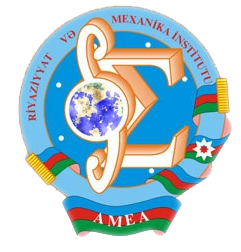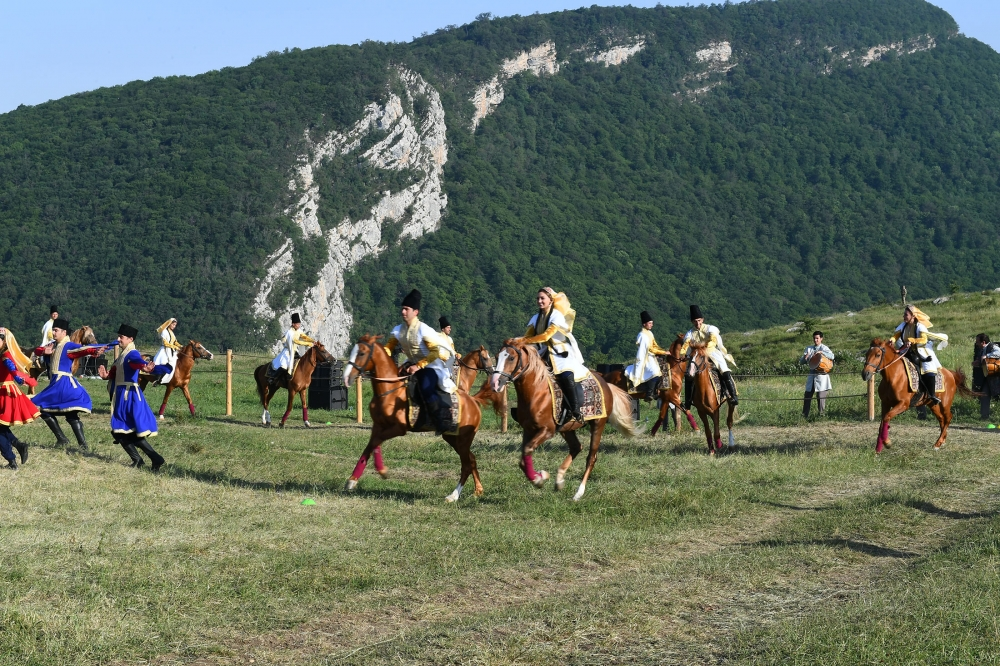Riyaziyyat və Mexanika İnstitutu

## Qarabağ xəbərləri### Şuşada Heydər Əliyev Fondunun təşkilatçılığı ilə “Musiqi irsi və Qarabağ atları Cıdır düzündə” adlı kompozisiya təqdim olunub## Transactions 2000/4/XX

 Year: 2000 Volume: 4 Number: XX About: [PDF] MATHEMATICS Aliyev M.J. Abstract [PDF] On Lp solvability in weighted spaces of the first boundary value problem for the Laplace equation in a domain with a conic point on the boundary. [PDF] 3 Amirov R.Kh. Abstract [PDF] Inverse problem for the Sturm-Liouville operator according to a spectrum and normalizing. [PDF] 11 Amirova L.I. Abstract [PDF] On the completeness of a part of eigen and adjoint vectors of a second order operator boundless class [PDF] 23 Aslanova N.M. Abstract [PDF] Ibe stability of the inverse problem of the scattering theory for non-self-adjoint operator [PDF] 30 Bandaliev R.A. Abstract [PDF] Imbedding theorems for anisotropic Banach-valued Sobolev spaces [PDF] 35 Gadjiev T.S., Aliyev S.Y. Abstract [PDF] On the behavior of solutions of non-linear parabolic equations in non-regular domains [PDF] 42 Gadjieva E.A., Gasanova T.Kh. Abstract [PDF] Approximation by two dimensional Bernstein-Chlodowsky polynomials in triangle with mobile boundary. [PDF] 47 Gadziyev A.I. Abstract [PDF] On fractional maximal functions and fractional integrals, generated by Bessel differential operators [PDF] 52 Garakhanova N.N. Abstract [PDF] The boundedness of Вкя maximal functions in spaces Ly’p” \Rk + j. [PDF] 64 Gulmamedov V.R.. Abstract [PDF] On some properties of boundary value problems for operator-differential equations on semi-axis. [PDF] 73 Ibadov N.V. Abstract [PDF] Convolution equations in spaces of functions of given increase near the boundary, II. Countable systems of inhomogeneous convolution equations. [PDF] 80 IPyasov N.A. Abstract [PDF] On approximation of multivariable periodic functions in the Я^т[й)] spaces. [PDF] 91 Iskenderovn.Sh. Abstract [PDF] The inverse scattering problem on a semi-axis for first order ordinary differential equations system. [PDF] 97 Ismailov M.I. Abstract [PDF] The inverse scattering problem for the first order symmetric hyperbolic equations system on the whole axis. [PDF] 109 Karatash H., lsmailov Z. Abstract [PDF] On a class of first order normal differential operators. [PDF] 115 Khankishiyev Z.F. Abstract [PDF] The solution of one difference problem for the equation of vibration of a stick with the separated variables method. [PDF] 123 Khanmamedov Ag.Kh. Abstract [PDF] On the theory of inverse scattering problems for a system of’difference equations.[PDF] 132 Mamedov l.T. Abstract [PDF] Strong solvability of the Dirichlet problem fornon-uniformly degenerate second order elliptic equations. [PDF] 136 Mukhtarov O.Sh., Kandemir Mustafa. Abstract [PDF] Asymptotic behavior of eigenvalues for the discontinuous problem with functionals in the boundary conditions. [PDF] 151 Mushlagov F.M. Abstract [PDF] Regularity of boundary points with respect to the first boundary value problem for degenerate parabolic equations. [PDF] 162 Novruzov E.B. Abstract [PDF] On properties of the solution of one non-linear parabolic equation. [PDF] 174 Salimov A.A., Magden A. Abstract [PDF] Horizontal lifts of tensor fields of type (1,1). [PDF] 182 МАП Sarigol, Jafarov S.Z. Abstract [PDF] Approximation classes of functions on R and И type continuums. [PDF] 192 Vahabov N.G. Abstract [PDF] The localization of spectrum and its application. [PDF] 202 Yagubova Kh.V. Abstract [PDF] On properties of the solution of one non-linear parabolic equation. [PDF] 215 MECHANICS Abdullayeva J.N. Abstract [PDF] The lorsional vibrations of a non-linear bioelastic round bar [PDF] 223 Kerimov Z.G., Fataliyev V.M. Abstract [PDF] Dynamics of planetary reductor [PDF] 227 Akbarov S.D., Soltanova S.M. Abstract [PDF] On determination of stress condition in the infinite anisotrop body containing two neighbouring periodically curved fibers [PDF] 233 APPLIED PROBLEMS OF MATHEMATICS AND MECHANICS Kerimov M.Z., Huseynov M.A. Abstract [PDF] Software engineering for setting conditions of gas-lift well operation [PDF] 239 Salyamova K.D., Khusanov B.E. Abstract [PDF] Electrokinetic effects in porous media as charge interaction display [PDF] 244 Tagiyev R.K. Abstract [PDF] On optimal control of the coefficients of the parabolic equation [PDF] 251 ANNIVERSARIES Babaev Melik-Bakhysh Ali-Ikram oglu [PDF] 258 Guseynov RaufVeli ogiu Mamedkhanov [PDF] 260 Jaraal Islam oglu [PDF] 262
Azərbaycanda COVID-19 ilə bağlı statistika
• Virusa yoluxan

599713

• Sağalan

569238

• Yeni yoluxan

1210

• Aktiv xəstə

22456

• Ölüm halı

8019

• Test edilib

5,633,054